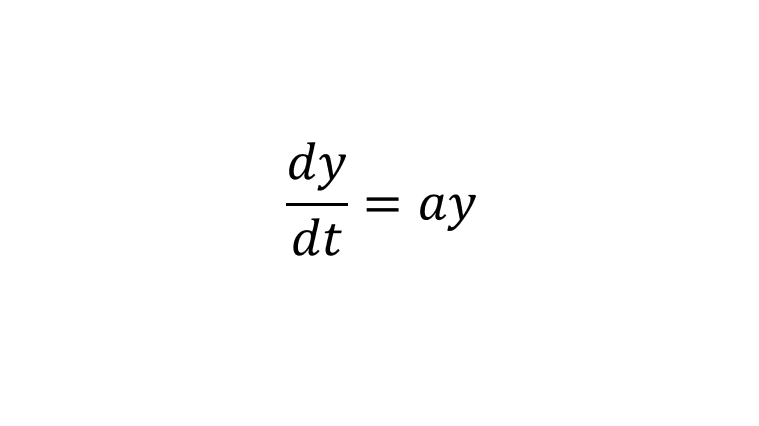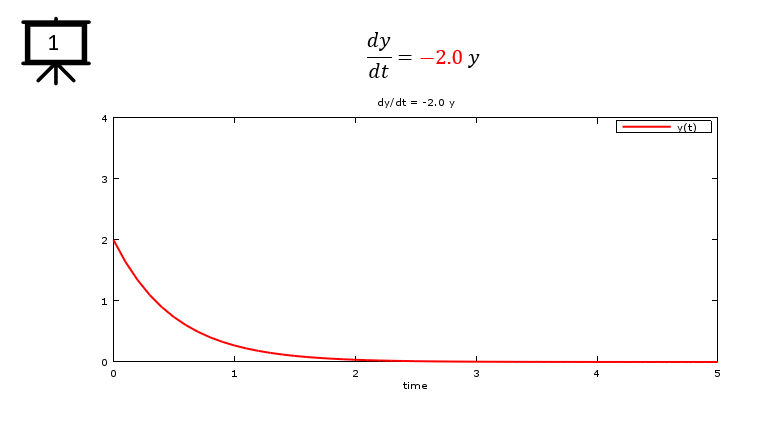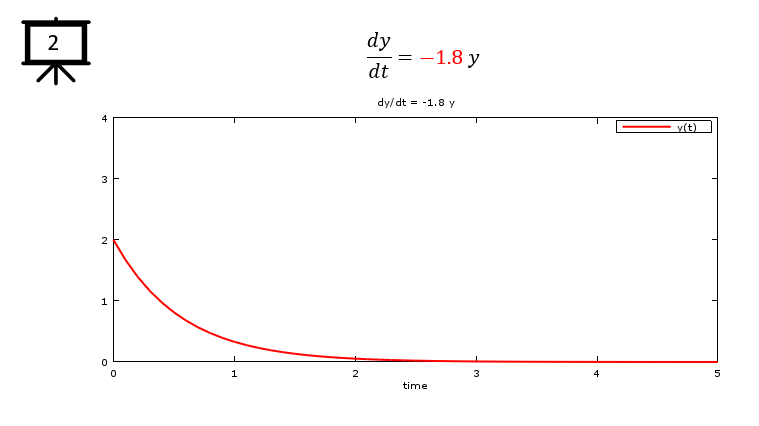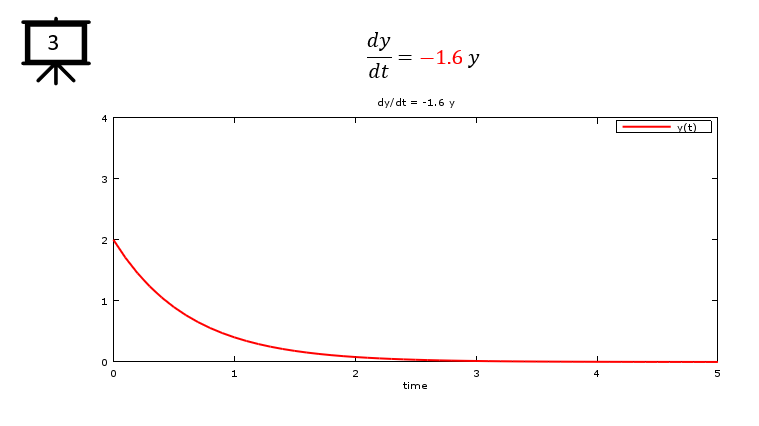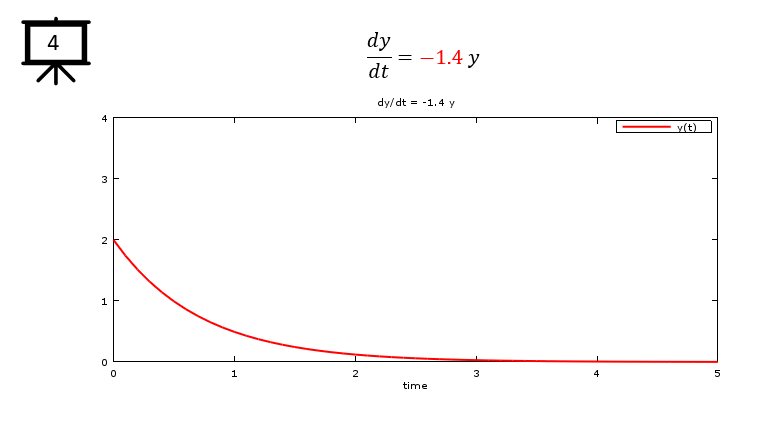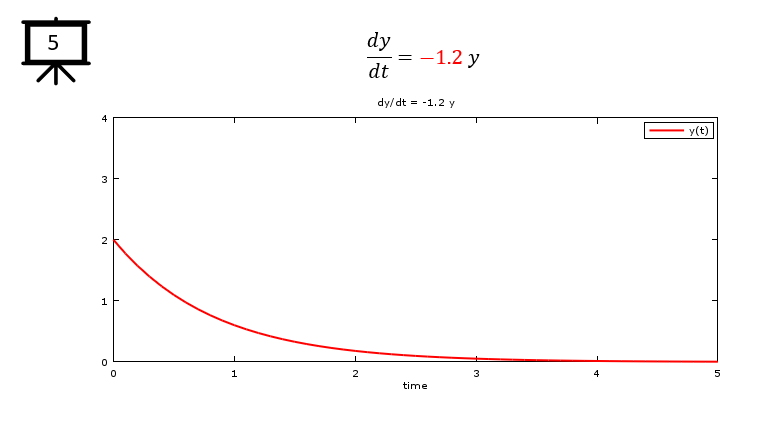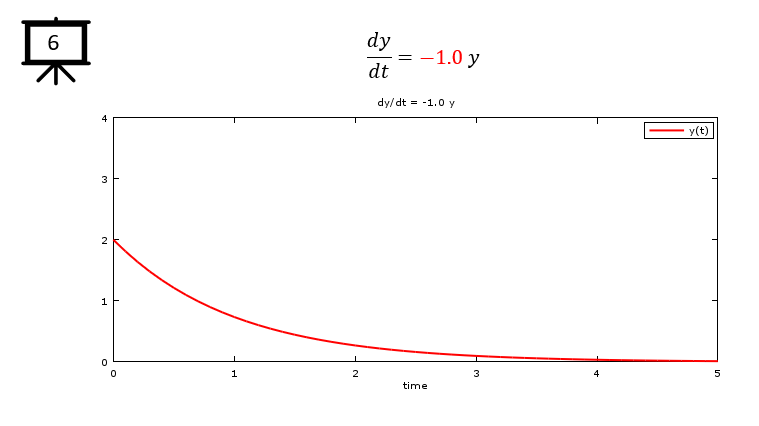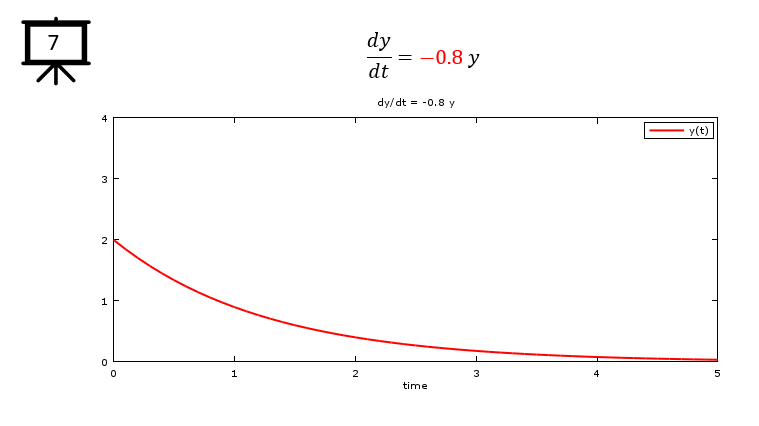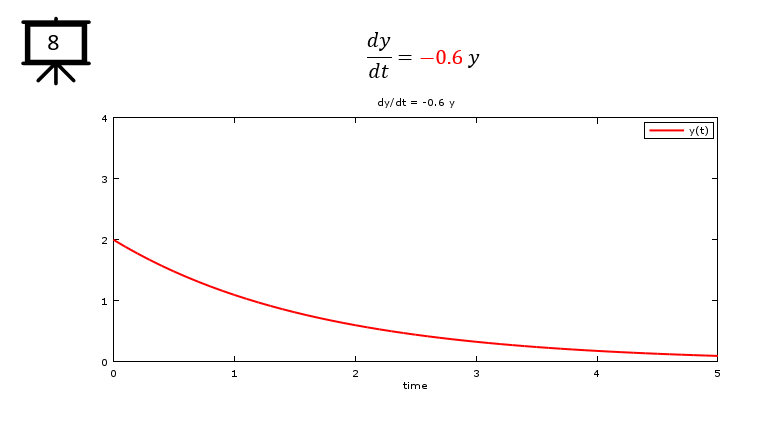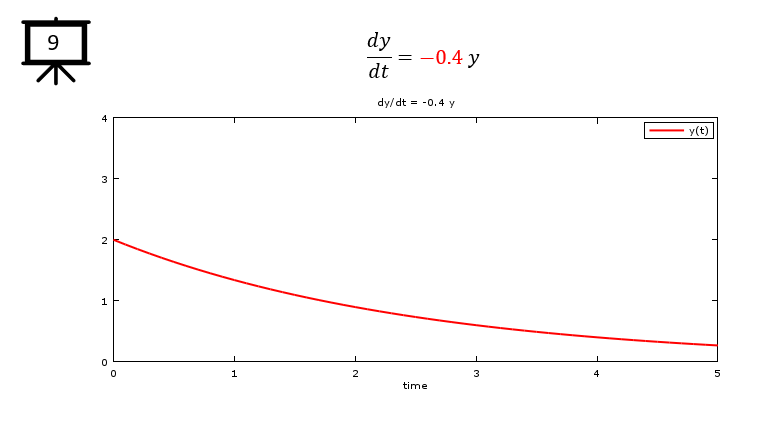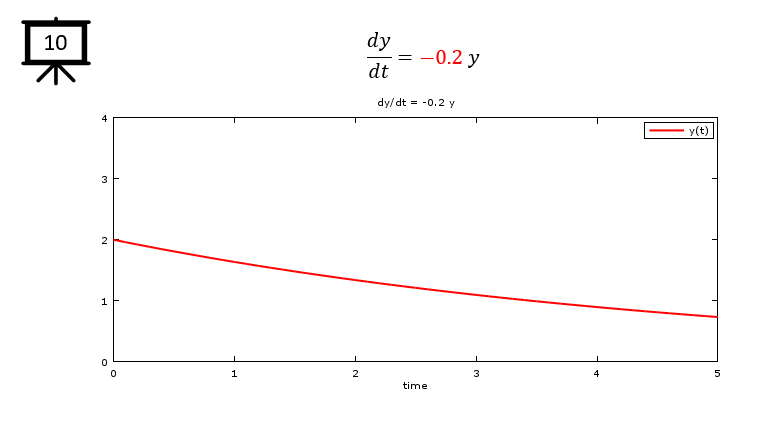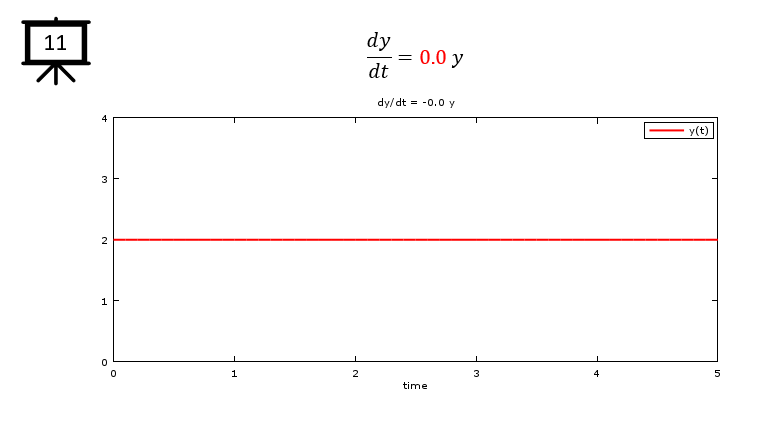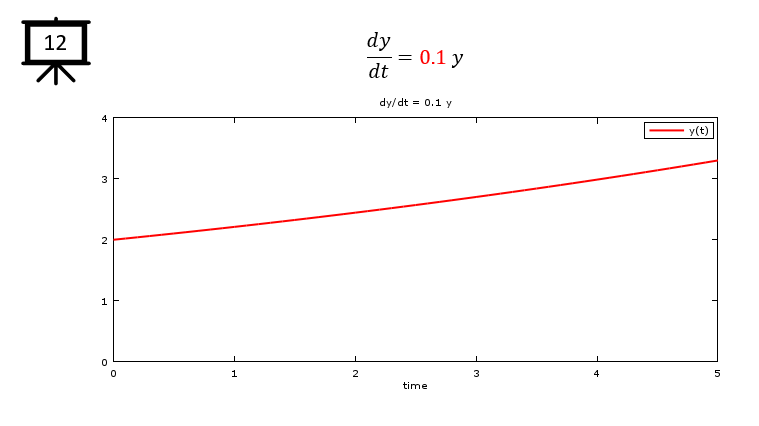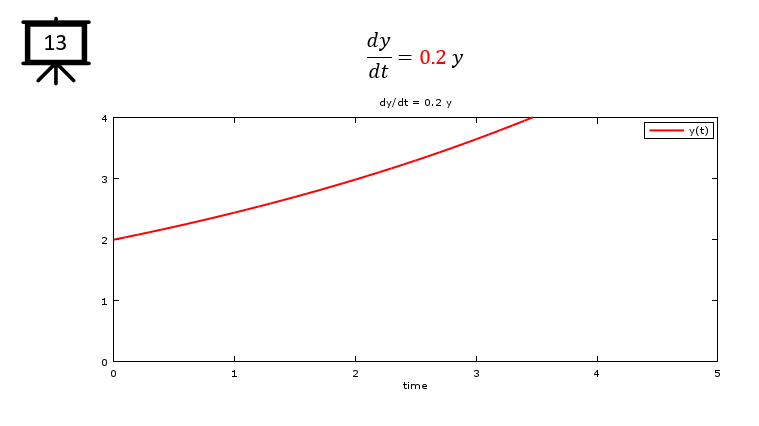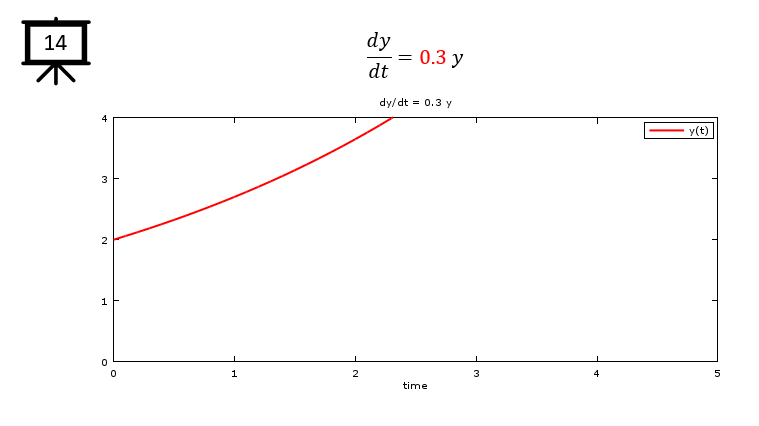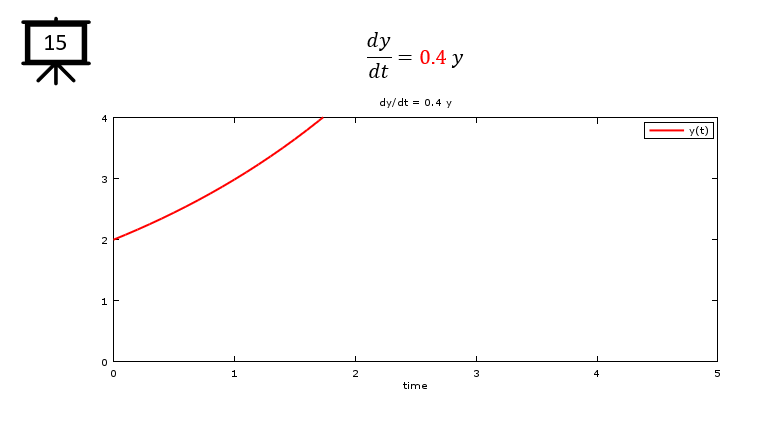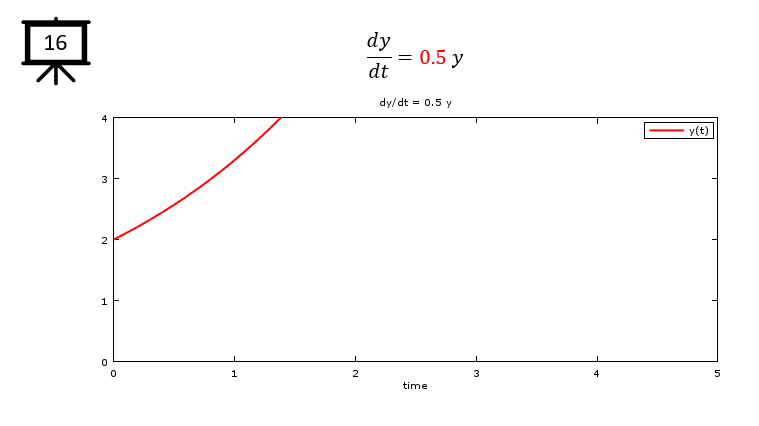1st Order Ordinary Differential Equation

 Followings are the code that I wrote in Octave to creates all the plots shown in this page. You may copy these code and play with these codes. Change variables and try yourself until you get your own intuitive understanding.   < Code 1 >   clear;   t = 0:0.1:5.0; global a = 0.5;    function dy_dt = f(y, t)   global a;   dy_dt = zeros (1,1);   dy_dt(1) = a .* y(1); endfunction   y0 = [2.0]; y = lsode ("f", y0, t);   tStr = sprintf("dy/dt = %0.1f y",a);   hFig = figure(1); plot(t,y(:,1),'r-','LineWidth',2); xlabel('time'); xlim([0 t(end)]); ylim([0 4]); legend('y(t)'); title(tStr);     set(hFig,'Position',[300 300 780 300]);